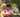### Binary Search

January 18, 2020

Binary Search in JavaScript

Binary Search is searching technique which works on Divide and Conquer approach. It used to search any element in a sorted array. As compared to linear, binary search is much faster with Time Complexity of O(logN) whereas linear search algorithm works in O(N) time complexity.

In each step, the algorithm compares the input element x with the value of the middle element in array. If the values match, return the index of middle. Otherwise, if x is less than the middle element, then the algorithm recurs for left side of middle element, else recurs for right side of middle element.

### Recursive Binary Search

``````const bs_recursive = (arr, target, start, end) => {
// Base Condition
if (start > end) return false;
// Find the middle index
let mid=Math.floor((start + end)/2);
// Compare mid with given key target
if (arr[mid]===target) return true;
// If element at mid is greater than target,
// search in the left half of mid
if(arr[mid] > target)
return bs_recursive(arr, target, start, mid-1);
else
// If element at mid is smaller than target,
// search in the right half of mid
return bs_recursive(arr, target, mid+1, end);
} ``````

### Iterative Binary Search

``````const bs_iterative = (arr, x) => {
let start=0, end=arr.length-1;
// Iterate while start not meets end
while (start<=end){
// Find the mid index
let mid=Math.floor((start + end)/2);
// If element is present at mid, return True
if (arr[mid]===x) return true;
// Else look in left or right half accordingly
else if (arr[mid] < x)
start = mid + 1;
else
end = mid - 1;
}
return false;
} ``````

### Binary Search Function

``````function bsearch(A, target){
console.log("\nsource: ", A)
A.sort((a,b)=>a-b) // must use sorted array for binary search
console.log("sorted: ", A)
console.log("target: ", target, " ,bs_recursive: ", bs_recursive(A, target, 0, A.length-1))
console.log("target: ", target, " ,bs_iterative: ", bs_iterative(A, target))
}
const arr = [7, 3, 1, 5, 8, 9]
const target1 = 5
const target2 = 6
bsearch(arr, target1)
bsearch(arr, target2)``````

Output:
source: [ 7, 3, 1, 5, 8, 9 ]
sorted: [ 1, 3, 5, 7, 8, 9 ]
target: 5 ,bs_recursive: true
target: 5 ,bs_iterative: true

source: [ 1, 3, 5, 7, 8, 9 ]
sorted: [ 1, 3, 5, 7, 8, 9 ]
target: 6 ,bs_recursive: false
target: 6 ,bs_iterative: falsePosted by Tai Lu ( 呂台生 ) who's building useful things.You should follow him on Twitter